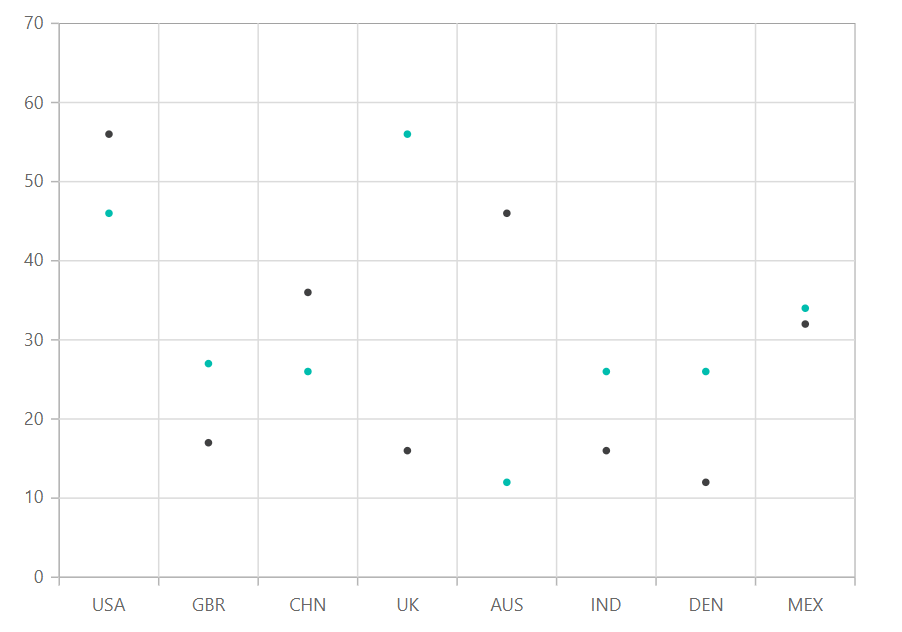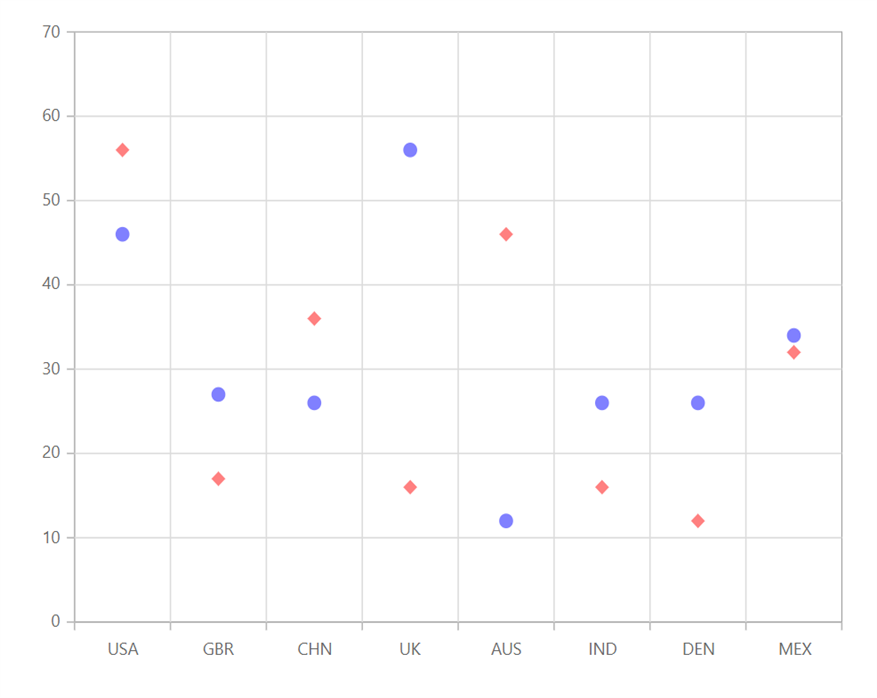Scatter in Blazor Charts Component

8 Dec 20214 minutes to read

Scatter

Scatter Chart is used to visualize the relationship between two Cartesian parameters. To render a Scatter Chart, set the series Type as Scatter.

@using Syncfusion.Blazor.Charts

<SfChart DataSource="@MedalDetails">
<ChartPrimaryXAxis ValueType="Syncfusion.Blazor.Charts.ValueType.Category"></ChartPrimaryXAxis>

<ChartSeriesCollection>
<ChartSeries XName="X" YName="YValue" Type="ChartSeriesType.Scatter">
</ChartSeries>
<ChartSeries XName="X" YName="YValue1" Type="ChartSeriesType.Scatter">
</ChartSeries>
</ChartSeriesCollection>
</SfChart>

@code{
public class ChartData
{
public string X { get; set; }
public double YValue { get; set; }
public double YValue1 { get; set; }
}

public List<ChartData> MedalDetails = new List<ChartData>
{
new ChartData { X= "USA", YValue= 46, YValue1=56 },
new ChartData { X= "GBR", YValue= 27, YValue1=17 },
new ChartData { X= "CHN", YValue= 26, YValue1=36 },
new ChartData { X= "UK", YValue= 56,  YValue1=16 },
new ChartData { X= "AUS", YValue= 12, YValue1=46 },
new ChartData { X= "IND", YValue= 26, YValue1=16 },
new ChartData { X= "DEN", YValue= 26, YValue1=12 },
new ChartData { X= "MEX", YValue= 34, YValue1=32},
};
}Refer to our Blazor Scatter Chart feature tour page to know about its other groundbreaking feature representations. Explore our Blazor Scatter Chart Example to know how to plot data with two numeric parameters.

Series Customization

The following properties can be used to customize the Scatter series.

• Fill – Specifies the color of the series.
• Opacity – Specifies the opacity of Fill.
• Shape - Specifies the shape of the scatter series.
@using Syncfusion.Blazor.Charts

<SfChart DataSource="@MedalDetails">
<ChartPrimaryXAxis ValueType="Syncfusion.Blazor.Charts.ValueType.Category"></ChartPrimaryXAxis>

<ChartSeriesCollection>
<ChartSeries XName="X" YName="YValue" Type="ChartSeriesType.Scatter" Fill="blue" Opacity="0.5">
<ChartMarker Height="10" Width="10" Shape="ChartShape.Circle">
</ChartMarker>
</ChartSeries>
<ChartSeries XName="X" YName="YValue1" Type="ChartSeriesType.Scatter" Fill="red" Opacity="0.5">
<ChartMarker Height="10" Width="10" Shape="ChartShape.Diamond">
</ChartMarker>
</ChartSeries>
</ChartSeriesCollection>
</SfChart>

@code{
public class ChartData
{
public string X { get; set; }
public double YValue { get; set; }
public double YValue1 { get; set; }
}

public List<ChartData> MedalDetails = new List<ChartData>
{
new ChartData { X= "USA", YValue= 46, YValue1=56 },
new ChartData { X= "GBR", YValue= 27, YValue1=17 },
new ChartData { X= "CHN", YValue= 26, YValue1=36 },
new ChartData { X= "UK", YValue= 56,  YValue1=16 },
new ChartData { X= "AUS", YValue= 12, YValue1=46 },
new ChartData { X= "IND", YValue= 26, YValue1=16 },
new ChartData { X= "DEN", YValue= 26, YValue1=12 },
new ChartData { X= "MEX", YValue= 34, YValue1=32},
};
}Refer to our Blazor Charts feature tour page for its groundbreaking feature representations and also explore our Blazor Chart Example to know various chart types and how to represent time-dependent data, showing trends at equal intervals.